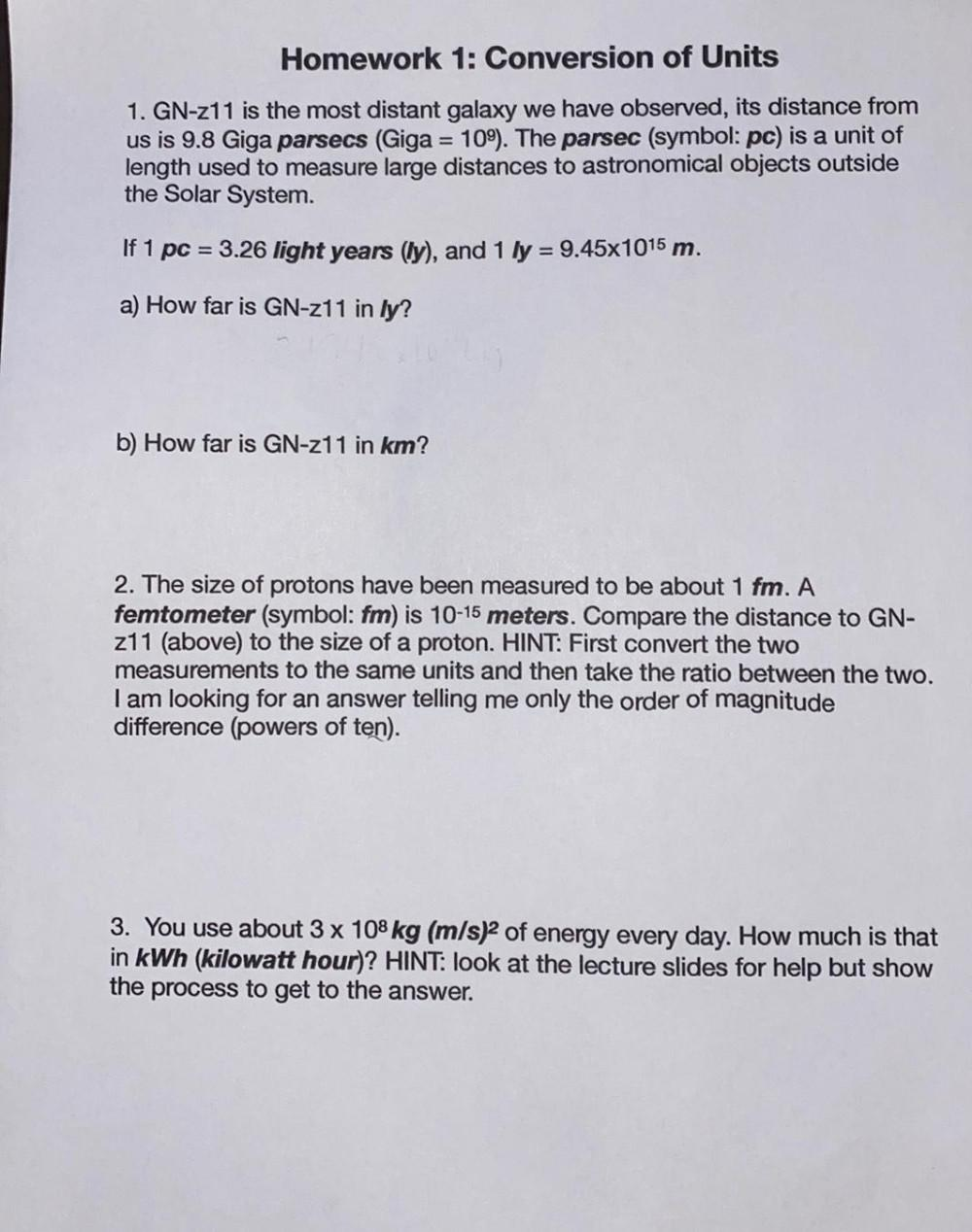Question:

# Homework 1: Conversion of Units 1. GN-z11 is the most distant galaxy we have observed, its distance from us is 9.8 Giga parsecsHomework 1: Conversion of Units 1. GN-z11 is the most distant galaxy we have observed, its distance from us is 9.8 Giga parsecs (Giga = 109). The parsec (symbol: pc) is a unit of length used to measure large distances to astronomical objects outside the Solar System. If 1 pc = 3.26 light years (ly), and 1 ly = 9.45x1015 m. a) How far is GN-z11 in ly? b) How far is GN-z11 in km? 2. The size of protons have been measured to be about 1 fm. A femtometer (symbol: fm) is 10-15 meters. Compare the distance to GN- z11 (above) to the size of a proton. HINT: First convert the two measurements to the same units and then take the ratio between the two. I am looking for an answer telling me only the order of magnitude difference (powers of ten). 3. You use about 3 x 108 kg (m/s)2 of energy every day. How much is that in kWh (kilowatt hour)? HINT: look at the lecture slides for help but show the process to get to the answer.